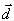##### Motion

There are two types of motion:

• Constant (Uniform) Motion (no acceleration - constant speed)
• Accelerated Motion (motion at changing speed)

Constant Motion obeys two conditions.  To determine if an object is in constant motion, both these conditions must be met:

1. Motion must be in a straight line
2. Motion must occur at constant speed

Examples:

• The seconds' hand on a clock is moving at constant speed but because it is revolving in a curved path, it cannot be considered constant motion.
• An apple falling from a tree falls in a straight line but because its speed is increasing due to gravitational  acceleration, it is not undergoing constant motion.
###### Accelerated Motion
1. Motion does not necessarily occurr in a straight line
2. The speed of the object changes over time intervals

4. Distance and Speed,

Distance is the change in position from an original point.  The symbol for distance is "d".
Distance is a scalar quantity because it does not take into account direction.

Example:

You go out of the front door of your house and start walking on the sidewalk.   You walk 10m and you decide to turn around and go back home along the same path, you will have traveled a total distance of 20 meters.  However, your total displacement will be zero meters.

Displacement is defined as the distance traveled in one direction.  The symbol for displacement isDisplacement is  a vector quantity.  [You can review about vectors here Vectors - review ]. The base unit for both displacement and distance is the meter (m).